## NCERT Solutions for Class 8 Maths Chapter 6 Squares and Square Roots Ex 6.3

NCERT Solutions for Class 8 Maths Chapter 6 Squares and Square Roots Ex 6.3 are part of NCERT Solutions for Class 8 Maths. Here we have given NCERT Solutions for Class 8 Maths Chapter 6 Squares and Square Roots Ex 6.3.

 Board CBSE Textbook NCERT Class Class 8 Subject Maths Chapter Chapter 6 Chapter Name Squares and Square Roots Exercise Ex 6.3 Number of Questions Solved 10 Category NCERT Solutions

## NCERT Solutions for Class 8 Maths Chapter 6 Squares and Square Roots Ex 6.3

Question 1.
What could be the possible ‘one’s’ digits of the square root of each of the following numbers ?
(i) 9801
(ii) 99856
(iii) 998001
(iv) 657666025.
Solution.
(i) 9801
∵ 1 x 1 = 1 and 9 x 9 = 81
∵ The possible one’s digit of the square root of the number 9801 could be 1 or 9.

(ii) 99856
∵ 4 x 4 = 16 and 6 x 6 = 36
∵ The possible one’s digit of the square root of the number 99856 could be 4 or 6.

(iii) 998001
∵ 1×1 = 1 and 9 x 9 = 81
∵ The possible one’s digit of the square root of the number 998001 could be 1 or 9.

(iv) 657666025
∵ 5 x 5 = 25
∵ The possible one’s digit of the square root of the number 657666025 could be 5.

Question 2.
Without doing any calculation, find the numbers which are surely not perfect squares.
(i) 153
(ii) 257
(iii) 408
Solution.
(i) 153
The number 153 is surely not a perfect square because it ends in 3 whereas the square numbers end with 0, 1, 4, 5, 6 or 9.

(ii) 257
The number 257 is surely not a perfect square because it ends in 7 whereas the square numbers end with 0, 1, 4, 5, 6 or 9.

(iii) 408
The number 408 is surely not a perfect square because it ends in 8 whereas the square numbers end with 0, 1, 4, 5, 6 or 9.

(iv) 441
The number may be a perfect square as the square numbers end wTith 0, 1, 4, 5, 6 or 9.

Question 3.
Find the square roots of 100 and 169 by the method of repeated subtraction.
Solution.
(i) 100

• 100 – 1 = 99
• 99 – 3 = 96
• 96 – 5 = 91
• 91 – 7 = 84
• 84 – 9 = 75
• 75 – 11 = 64
• 64 – 13 = 51
• 51 – 15 = 36
• 36 – 17 = 19
• 19 – 19 = 0

Since from 100, we subtracted successive odd numbers starting from 1 and obtained 0 at the 10th step, therefore,
$$\sqrt { 100 } =10$$

(ii) 169

• 169 – 1 = 168
• 168 – 3 = 165
• 165 – 5 = 160
• 160 – 7 = 153
• 153 – 9 = 144
• 144-11 = 133
• 133 – 13 = 120
• 120 – 15 = 105
• 105 – 17 = 88
• 88 – 19 = 69
• 69 – 21 = 48
• 48 – 23 = 25
• 25 – 25 = 0

Since rom 169, we subtracted successive odd numbers starting from 1 and obtained 0 at the 13th step, therefore,
$$\sqrt { 169 } =13$$

Question 4.
Find the square roots of the following numbers by the Prime Factorisation Method:
(i) 729
(ii) 400
(iii) 1764
(iv) 4095
(v) 7744
(vi) 9604
(vii) 5929
(viii) 9216
(ix) 529
(x) 8100
Solution.
(i) 729
The prime factorisation of 729 is
729 = 3 x 3 x 3 x 3 x 3 x 3.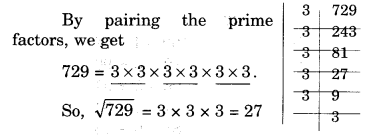(ii) 400
The prime factorisation of 400 is
400 = 2 x 2 x 2 x 2 x 5 x 5.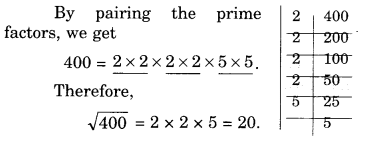(iii) 1764(iv) 4096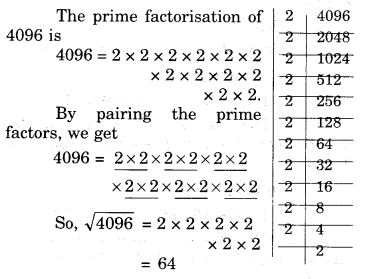(v) 7744(vi) 9604(vii) 5929(viii) 9216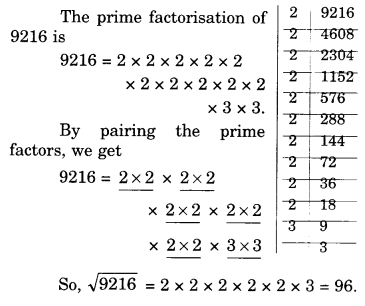(ix) 529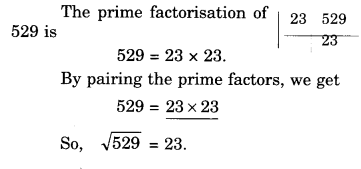(x) 8100Question 5.
For each of the following numbers, find the smallest whole number by which it should be multiplied so as to get a perfect square number. Also find the square root of the square number so obtained.
(i) 252
(ii) 180
(iii) 1008
(iv) 2028
(v) 1458
(vi) 768
Solution.
(i) 252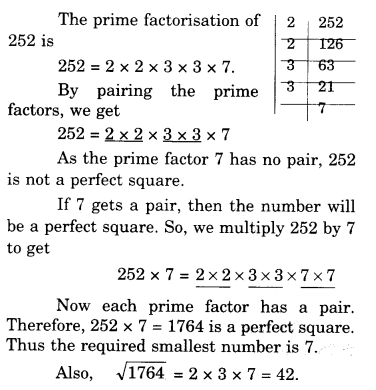(ii) 180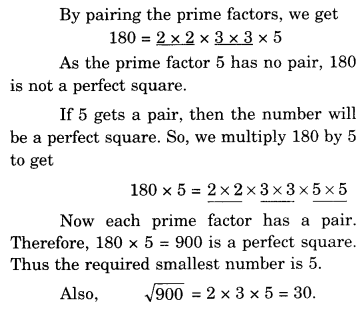(iii) 1008(iv) 2028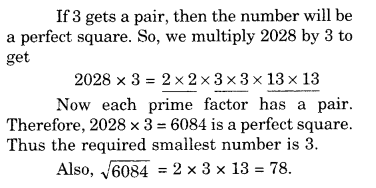(v) 1458(vi) 768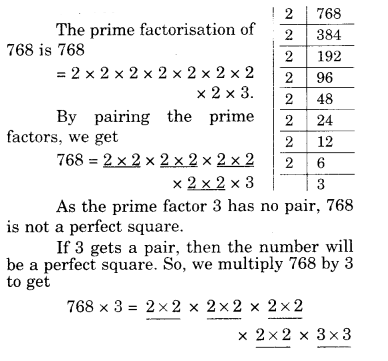Question 6.
For each of the following numbers, find the smallest whole number by which it should be divided so as to get a perfect square. Also find the square root of the square number so obtained.
(i) 252
(ii) 2925
(iii) 396
(iv) 2645
(v) 2800
(vi) 1620
Solution.
(i) 252(ii) 2925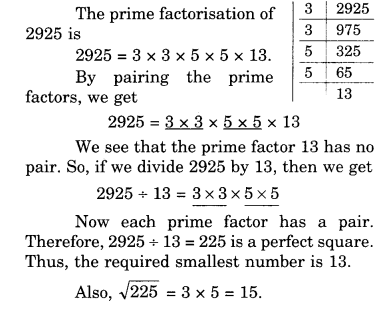(iii) 396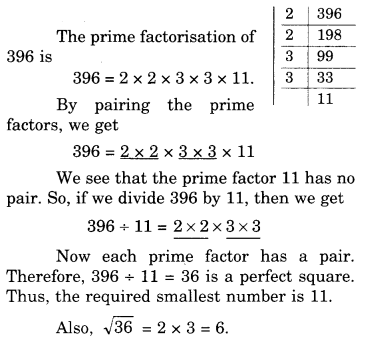(iv) 2645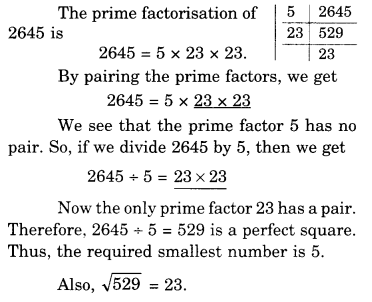(v) 2800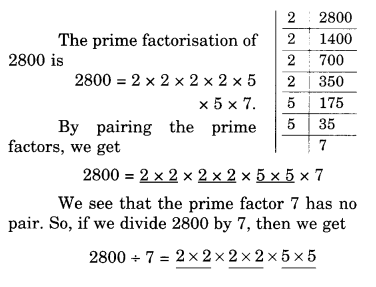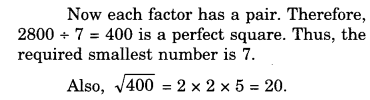(vi) 1620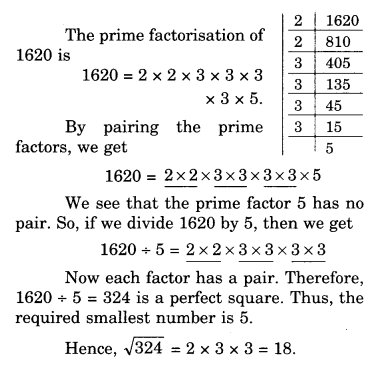Question 7.
The students of Class VIII of a school donated? 2401 in all, for Prime Minister’s National Relief Fund. Each student donated as rr’iny rupees as the number of students in the class. Find the number of students in the class.
Solution.
Let the number of students in the class be x.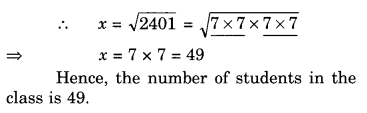Question 8.
2025 plants are to be planted in a garden in such a way that each row contains as many plants as the number of rows. Find the number of rows and the number of plants in each row.
Solution.
Let the number of rows be x.
Then, number of plants in each row = x.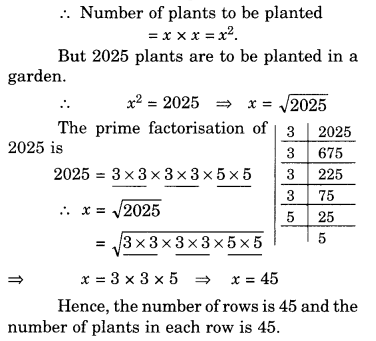Question 9.
Find the smallest square number that is divisible by each of the numbers 4, 9 and 10.
Solution.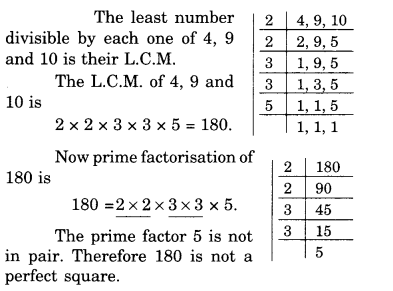In order to get a perfect square, each factor of 180 must be paired. So, we need to make pair of 5.
Therefore, 180 should be multiplied by 5.
Hence, the required smallest square number is 180 x 5 = 900.

Question 10.
Find the smallest square number that is divisible by each of the numbers 8, 15 and 20.
Solution.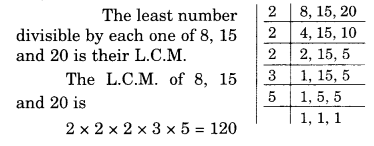We hope the NCERT Solutions for Class 8 Maths Chapter 6 Squares and Square Roots Ex 6.3 are part help you. If you have any query regarding NCERT Solutions for Class 8 Maths Chapter 6 Squares and Square Roots Ex 6.3 are part, drop a comment below and we will get back to you at the earliest.

## NCERT Solutions for Class 8 Maths Chapter 6 Squares and Square Roots Ex 6.2

NCERT Solutions for Class 8 Maths Chapter 6 Squares and Square Roots Ex 6.2 are part of NCERT Solutions for Class 8 Maths. Here we have given NCERT Solutions for Class 8 Maths Chapter 6 Squares and Square Roots Ex 6.2.

 Board CBSE Textbook NCERT Class Class 8 Subject Maths Chapter Chapter 6 Chapter Name Squares and Square Roots Exercise Ex 6.2 Number of Questions Solved 2 Category NCERT Solutions

## NCERT Solutions for Class 8 Maths Chapter 6 Squares and Square Roots Ex 6.2

Question 1.
Find the square of the following numbers:
(i) 32
(ii) 35
(iii) 86
(iv) 93
(v) 71
(vi) 46
Solution.
(i) 32
32 = 30 + 2
Therefore, $${ 32 }^{ 2 }$$ = $${ \left( 30+2 \right) }^{ 2 }$$
= 30 (30 + 2) + 2 (30 + 2)
= 900 + 60 + 60 + 4
= 1024

(ii) 35
35 = 30 + 5
Therefore, $${ 35 }^{ 2 }$$ = $${ \left( 30+5 \right) }^{ 2 }$$
= 30 (30 + 5) + 5 (30 + 5)
= 900 + 150 + 150 + 25
= 1225

(iii) 86
86 = 80 + 6
Therefore, $${ 86 }^{ 2 }$$= $${ \left( 80+6 \right) }^{ 2 }$$
= 80 (80 + 6) + 6 (80 + 6)
= 6400 + 480 + 480 + 36
= 7396

(iv) 93
93 = 90 + 3
Therefore, $${ 90 }^{ 2 }$$= $${ \left( 90+3\right) }^{ 2 }$$
= 90 (90 + 3) + 3 (90 + 3)
= 8100 + 270 + 270 + 9
= 8649

(v) 71
71 = 70 + 1
Therefore, $${ 70 }^{ 2 }$$= $${ \left( 70+1\right) }^{ 2 }$$
= 70 (70 + 1) + 1 (70 + 1)
= 4900 + 70 + 70 + 1
= 5041

(vi) 46
46 = 40 + 6
Therefore, $${ 40 }^{ 2 }$$= $${ \left( 40+6\right) }^{ 2 }$$
= 40 (40 + 6) + 6 (40 + 6)
= 1600 + 240 + 240 + 36
= 2116

Question 2.
Write a Pythagorean triplet whose one number is
(i) 6
(ii) 14
(iii) 16
(iv) 18
Solution.
(i) 6
Let 2m = 6
⇒ $$m=\frac { 6 }{ 2 } =3$$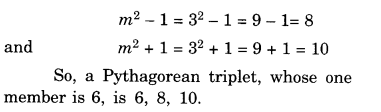(ii) 14
Let 2m = 14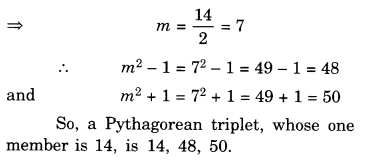(iii) 16
Let 2m = 16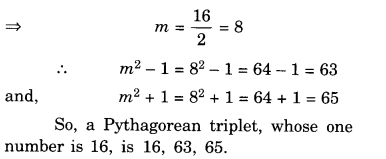(iv) 18
Let 2m = 18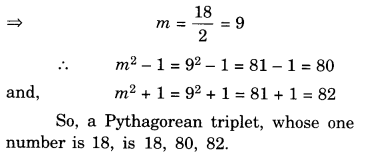We hope the NCERT Solutions for Class 8 Maths Chapter 6 Squares and Square Roots Ex 6.2 are part help you. If you have any query regarding NCERT Solutions for Class 8 Maths Chapter 6 Squares and Square Roots Ex 6.2 are part, drop a comment below and we will get back to you at the earliest.

## NCERT Solutions for Class 8 Maths Chapter 5 Data Handling Ex 5.3

NCERT Solutions for Class 8 Maths Chapter 5 Data Handling Ex 5.3 are part of NCERT Solutions for Class 8 Maths. Here we have given NCERT Solutions for Class 8 Maths Chapter 5 Data Handling Ex 5.3.

 Board CBSE Textbook NCERT Class Class 8 Subject Maths Chapter Chapter 5 Chapter Name Data Handling Exercise Ex 5.3 Number of Questions Solved 6 Category NCERT Solutions

## NCERT Solutions for Class 8 Maths Chapter 5 Data Handling Ex 5.3

Question 1.
List the outcomes you can see in these experiments.
(a) Spinning a wheel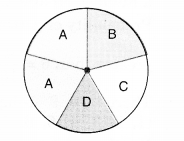(b) Tossing two coins together
Solution.
(a) Outcomes in spinning the given wheel are A, B, C and D.
(b) Outcomes in tossing two coins together are HT, HH, TH, TT (Here HT means Head on first coin and Tail on the second coin and so on).

Question 2.
When a die is thrown, list the outcomes of an event of getting
(i)
(a) a prime number
(b) not a prime number

(ii)
(a) a number greater than 5
(b) a number not greater than 5.
Solution.
Possible outcomes are:
1, 2, 3, 4, 5, and 6.
Out of these, prime numbers are
2, 3 and 5.

(i)
(a) Outcomes of an event of getting a prime number are: 2, 3 and 5
(b) Outcomes of an event of not getting a prime number are 1, 4 and 6.

(ii)
(a) Outcomes of an event of getting a number greater than 5 are 6
(b) Outcomes of an event of getting a; number not greater than 5 are 1, 2, 3, 4 and 5.

Question 3.
Find the
(a) Probability of the pointer stopping on D in (Question l-(a))?
(b) Probability of getting an ace from a j well shuffled deck of 52 playing cards?
(c) Probability of getting a red apple. (See figure)Solution.
(a) There are in all 5 outcomes of the event. These are A, B, C and D. The pointer stopping on D has only 1 outcome, i.e., D
∴ Probability of the pointer stopping on D =$$\frac { 1 }{ 5 }$$

(b) Total number of playing cards = 52 Number of possible outcomes = 52
Number of aces in a deck of playing cards = 4
cards = 4
∴ Probability of getting an ace from a well shuffled deck of 52
playing cards = $$\frac { 4 }{ 52 }$$ = $$\frac { 1 }{ 13 }$$

(c) Total number of apples = 7
Number of red apples = 4
∴ Probability of getting a red apple = $$\frac { 4 }{7}$$

Question 4.
Numbers 1 to 10 are written on ten separate slips (one number on one slip), kept in a box and mixed well. One slip is chosen from the box without looking into it. What is the probability of?
(i) getting a number 6?
(ii) getting a number less than 6?
(iii) getting a number greater than 6?
(iv) getting a 1-digit number?
Solution.
Total number of outcomes of the event (1, 2, 3, 4, 5, 6, 7, 8, 9 and 10) = 10
(i)
∵ Number of outcomes of getting a number 6=1
∴ Probability of getting a number $$\frac { 1 }{10}$$

(ii)
∵ There are 5 numbers (1, 2, 3, 4 and 5) less than 6.
∴ Number of outcomes of getting a number less than 6 = 5
∴ Probability of getting a number less than $$6=\frac { 5 }{ 10 } =\frac { 1 }{ 2 }$$

(iii)
∵ There are 4 numbers (7, 8, 9 and 10) greater than 6
∴ Number of outcomes of getting a number greater than 6 = 4
∴ Probability of getting a number greater than $$6=\frac { 4 }{ 10 } =\frac { 2}{ 15 }$$

(iv)
∵ There are 9 1-digit numbers (1, 2, 3, 4, 5, 6, 7, 8 and 9)
∴ Number of outcomes of getting a 1-digit number = 9
∴ Probability of getting a 1-digit number $$=\frac { 9 }{ 10 }$$

Question 5.
If you have a spinning wheel with 3 green sectors, 1 blue sector and 1 red sector, what is the probability of getting a green sector? What is the probability of getting a nonblue sector?
Solution.
Number of green sectors = 3
Number of blue sectors = 1
Number of red sectors = 1
∴ Total number of sectors = 3 + 1 + 1=5
∴ Total number of outcomes of the event = 5
Number of outcomes of getting a green sector = 3
∴ Probability of getting a green sector = $$\frac { 3 }{ 5 }$$
Number of outcomes of getting a non-blue sector = Number of green sectors + Number of red sectors
=3+1=4
∴ Probability of getting a non-blue sector = $$\frac { 4 }{ 5 }$$.

Question 6.
Find the probabilities of the events given in Question 2.
Solution.
Total number of outcomes of the event (1, 2, 3, 4, 5 and 6) = 6
(i)
(a) Number of prime numbers (2, 3 and 5) = 3
∴ Number of outcomes of getting a prime number = 3
∴ Probability of getting a prime number = $$\frac { 3 }{ 6 } =\frac { 1 }{ 2 }$$.
(b) Number of non-prime numbers (1, 4 and 6) = 3
∴ Number of outcomes of getting a non-prime number = 3
∴ Probability of getting a non-prime number = $$\frac { 3 }{ 6 } =\frac { 1 }{ 2 }$$.

(ii)
(a) Number greater than 5 = 6, i.e., only one.
∴ Number of outcomes of getting a number greater than 5 = 1
∴ Probability of getting a number greater than 5 = $$\frac { 1 }{ 6 }$$.
(b) Number of numbers not greater than 5 (1, 2, 3, 4 and 5) = 5
∴ Number of outcomes of getting a number not greater than 5 = 5
∴ Probability of getting a number not greater than 5 = $$\frac { 5 }{ 6 }$$.

We hope the NCERT Solutions for Class 8 Maths Chapter 5 Data Handling Ex 5.3 help you. If you have any query regarding NCERT Solutions for Class 8 Maths Chapter 5 Data Handling Ex 5.3, drop a comment below and we will get back to you at the earliest.

## NCERT Solutions for Class 8 Maths Chapter 5 Data Handling Ex 5.2

NCERT Solutions for Class 8 Maths Chapter 5 Data Handling Ex 5.2 are part of NCERT Solutions for Class 8 Maths. Here we have given NCERT Solutions for Class 8 Maths Chapter 5 Data Handling Ex 5.2.

 Board CBSE Textbook NCERT Class Class 8 Subject Maths Chapter Chapter 5 Chapter Name Data Handling Exercise Ex 5.2 Number of Questions Solved 5 Category NCERT Solutions

## NCERT Solutions for Class 8 Maths Chapter 5 Data Handling Ex 5.2

Question 1.
A survey was made to find the type of music that a certain group of young people liked in a city. The adjoining pie chart shows the findings of this survey.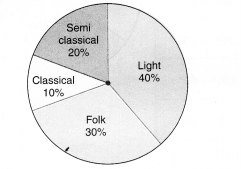From this pie chart answer the following:
(i) If 20 people liked classical music, how many young people were surveyed?
(ii) Which type of music is liked by the maximum number of people?
(iii) If a cassette company were to make 1000 CD’s, how many of each type would they make?
Solution.
(i) Suppose that x young people were surveyed. Then, the number of young people who liked classical music = 10% of x
$$\frac { 10 }{ 100 } \times x=\frac { x }{ 10 }$$According to the question,
$$\frac { x }{ 10 } =20$$
⇒ x = 20 x 10
⇒ x = 200
Hence, 200 young people were surveyed.

(ii) Light music is liked by the maximum number of people.

(iii) Total number of CD’s = 1000 Number of CD’s of Semi Classical music = 20% of 1000
⇒ $$\frac { 20 }{ 100 } \times 1000=200$$
Number of CD’s of Classical music = 10% of 1000
⇒ $$\frac { 10 }{ 100 } \times 1000=100$$
Number of CD’s of Folk music = 30% of 1000
⇒ $$\frac { 30 }{ 100 } \times 1000=300$$
Number of CD’s of Light music = 40% of 1000
⇒ $$\frac { 40 }{ 100 } \times 1000=400$$

Question 2.
A group of 360 people was asked to vote for their favorite season from the three seasons rainy, winter and summer.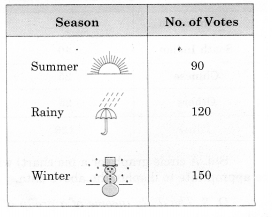(i) Which season got the most votes?
(ii) Find the central angle of each sector.
(iii) Draw a pie chart to show this information.
Solution.
(i) Winter season got the most votes.

(ii) Total votes = 90 + 120 + 150 = 360. Central angle of winter sector
$$= \frac { Number\quad of\quad people\quad who\quad vote\quad for\quad winter\quad season }{ Total\quad number\quad of\quad people } \times { 360 }^{ \circ }$$
= $$\frac { 150 }{ 360 } \times { 360 }^{ \circ }={ 150 }^{ \circ }$$
Central angle of summer sector
$$= \frac { Number\quad of\quad people\quad who\quad vote\quad for\quad summer\quad season }{ Total\quad number\quad of\quad people } \times { 360 }^{ \circ }$$
= $$\frac { 90 }{ 360 } \times { 360 }^{ \circ }={ 90 }^{ \circ }$$
Central angle of rainy sector
$$= \frac { Number\quad of\quad people\quad who\quad vote\quad for\quad rainy\quad season }{ Total\quad number\quad of\quad people } \times { 360 }^{ \circ }$$
= $$\frac { 120}{ 360 } \times { 360 }^{ \circ }={ 120 }^{ \circ }$$

(iii)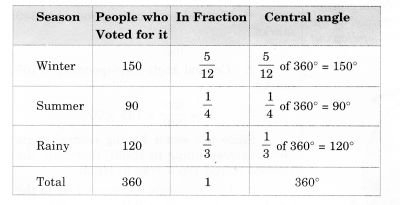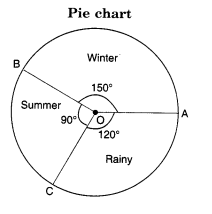Question 3.
Draw a pie chart showing the following information. The table shows the colors preferred by a group of people.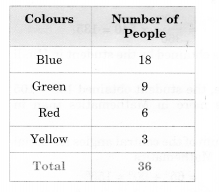Find the proportion of each sector. For example, Blue is $$\frac { 18 }{ 36 } =\frac { 1 }{ 2 }$$ ; Green is $$\frac { 9 }{ 36 } =\frac { 1 }{ 4 }$$ ; and so on. Use this to find the corresponding angles.
Solution.Question 4.
The adjoining pie chart gives the marks scored in an examination by a student in Hindi, English, Mathematics, Social Science and Science. If the total marks obtained by the students were 540, answer the following questions.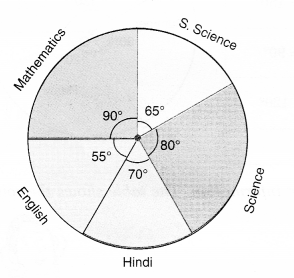(i) In which subject did the student score 105 marks?
(Hint: For 540 marks, the central angle = 360°. So, for 105 marks, what is the central angle ?)
(ii) How many more marks were obtained by the student in Mathematics than in Hindi?
(iii) Examine whether the sum of the marks obtained in Social Science and Mathematics is more than that in Science and Hindi.
(Hint; Just study the central angles).
Solution.
(i) Total marks = 540
∵ Central angle corresponding to 540 = 360°
∴ Central angle corresponding to 105
$$\frac { { 360 }^{ \circ } }{ 540 } \times \left( 105 \right) ={ 70 }^{ \circ }$$
Since the sector having central angle 70° is corresponding to Hindi, therefore, the student scored 105 marks in Hindi.

(ii) Central angle corresponding to the sector of Mathematics = 90°
∴ Marks obtained by the student in Mathematics
$$\frac { { 90 }^{ \circ } }{ { 360 }^{ \circ } } \times 540=135$$.
Marks obtained by the student in Hindi = 105.
Hence, the student obtained 135 – 105 = 30 marks more in Mathematics than in Hindi.

(iii) Sum of the central angles for Social Science and Mathematics
= 65° + 90° = 155°
Sum of the central angles for Science and Hindi
= 80° + 70° = 150°
Since the marks obtained are proportional to the central angles corresponding to various subjects and 155° > 150°, therefore the sum of the marks obtained in Social Science and Mathematics is more than that in Science and Hindi.

Question 5.
The number of students in a hostel, speaking different languages is given below. Display the data in a pie chart.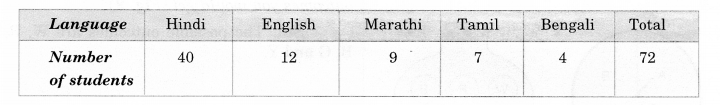Solution.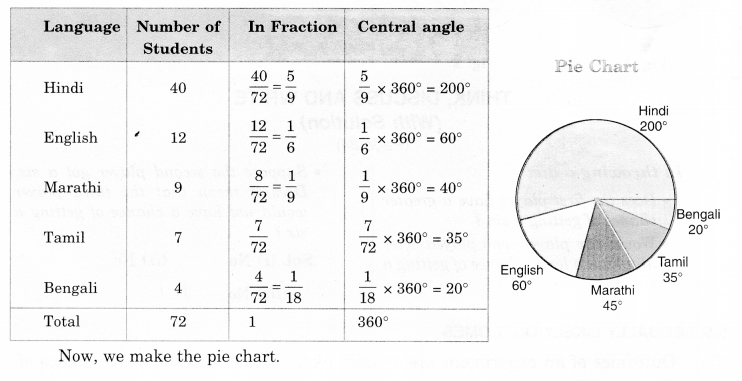We hope the NCERT Solutions for Class 8 Maths Chapter 5 Data Handling Ex 5.2 help you. If you have any query regarding NCERT Solutions for Class 8 Maths Chapter 5 Data Handling Ex 5.2, drop a comment below and we will get back to you at the earliest.

## NCERT Solutions for Class 8 Maths Chapter 4 Practical Geometry Ex 4.5

NCERT Solutions for Class 8 Maths Chapter 4 Practical Geometry Ex 4.5 are part of NCERT Solutions for Class 8 Maths. Here we have given NCERT Solutions for Class 8 Maths Chapter 4 Practical Geometry Ex 4.5.

 Board CBSE Textbook NCERT Class Class 8 Subject Maths Chapter Chapter 4 Chapter Name Practical Geometry Exercise Ex 4.5 Number of Questions Solved 1 Category NCERT Solutions

## NCERT Solutions for Class 8 Maths Chapter 4 Practical Geometry Ex 4.5

Question 1.
Draw the following:
1. The square READ with RE = 5.1 cm.
2. A rhombus whose diagonals are 5.2 cm and 6.4 cm long.
3. A rectangle with adjacent sides of lengths 5 cm and 4 cm.
4. A parallelogram OKAY where OK = 5.5 cm and KA = 4.2 cm. Is it unique?
Solution.
1. Steps of Construction

1. Draw RE = 5.1 cm.
2. At R, draw a ray RX such that ∠ERX3. From ray RX, cut RD = 5.1 cm.
4. At E, draw a ray EY such that ∠REY = 90°.
5. From ray EY, cut EA = 5.1 cm.

Then, READ is the required square.

2. Steps of Construction
[We know that the diagonals of a rhombus bisect each other at right angles. So in rhombus ABCD, the diagonals AC and BD will bisect each other at right angles.]

1. Draw AC = 5.2 cm.
2. Construct its perpendicular bisector. Let it intersect AC at O.3. Cut off $$\frac { 6.4 }{ 2 }$$= 3.2 cm lengths on either side of the bisector drawn in step 2, we get B and D.
4. Join AB, BC, CD, and DA.

Then, ABCD is the required rhombus.

3. Steps of Construction
[We know that each angle of a rectangle is 90°. So, in rectangle PQRS,
∠P=∠Q=∠R=∠S= 90°.
Also, opposite sides of a rectangle are parallel.
So, in rectangle PQRS,
PQ || SR and PS || QR]

1. Draw PQ = 5 cm.
2. At Q, draw a ray QX such that ∠PQX = 90°.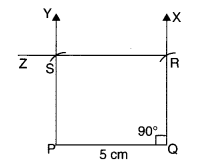3. From ray QX, cut QR = 4 cm.
4. At P, draw a ray PY parallel to QR.
5. At R, draw a ray RZ parallel to QP to meet the ray drawn in step 4 at S.

Then, PQRS is the required rectangle.

4. Steps of Construction
[We know that in a parallelogram, opposite sides are parallel and equal. So,
OK = YA and OK || YA;
KA = OY and KA || OY]

1. Draw OK = 5.5 cm.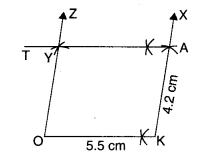2. At K, draw a ray KX at any suitable angle from OK.
3. From ray KX, cut KA = 4.2 cm.
4. A, draw a ray AT parallel to KO.
5. At O, draw a ray OZ parallel to KA to cut the ray drawn in step 4 at Y.

Then, OKAY is the required parallelogram.
This is not unique.
Note: We can construct countless parallelograms with these dimensions by varying ∠OKA

We hope the NCERT Solutions for Class 8 Maths Chapter 4 Practical Geometry Ex 4.5 help you. If you have any query regarding NCERT Solutions for Class 8 Maths Chapter 4 Practical Geometry Ex 4.5, drop a comment below and we will get back to you at the earliest.

## NCERT Solutions for Class 8 Maths Chapter 4 Practical Geometry Ex 4.4

NCERT Solutions for Class 8 Maths Chapter 4 Practical Geometry Ex 4.4 are part of NCERT Solutions for Class 8 Maths. Here we have given NCERT Solutions for Class 8 Maths Chapter 4 Practical Geometry Ex 4.4.

 Board CBSE Textbook NCERT Class Class 8 Subject Maths Chapter Chapter 4 Chapter Name Practical Geometry Exercise Ex 4.4 Number of Questions Solved 1 Category NCERT Solutions

## NCERT Solutions for Class 8 Maths Chapter 4 Practical Geometry Ex 4.4

Question 1.
DE = 4 cm
EA = 5 cm
AR = 4.5 cm
∠E = 60°
∠A = 90°

TR = 3.5 cm
RU = 3 cm
UE = 4 cm
∠R = 75°
∠U= 120°
Solution.
(i) Steps of Construction

1. Draw DE = 4 cm.
2. At E, draw ray EX such that ∠DEX = 60°.
3. From ray EX, cut EA = 5 cm.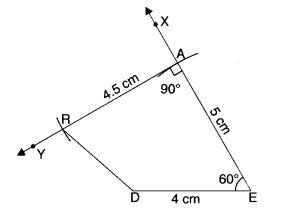4. At A, draw ray AY such that ∠EAY = 90°.
5. Cut AR = 4.5 cm from ray AY.
6. Join RD.

Then, DEAR is the required quadrilateral.

(ii) Steps of Construction

1. Draw TR = 3.5 cm.
2. At R, draw ray RX such that ∠TRX = 75°.3. Cut RU = 3 cm from ray RX.
4. At U, draw ray UY such that ∠RUY = 120°.
5. Cut UE = 4 cm from ray UY.
6. Join ET.

Then, TRUE is the required quadrilateral.

We hope the NCERT Solutions for Class 8 Maths Chapter 4 Practical Geometry Ex 4.4 help you. If you have any query regarding NCERT Solutions for Class 8 Maths Chapter 4 Practical Geometry Ex 4.4, drop a comment below and we will get back to you at the earliest.

## NCERT Solutions for Class 8 Maths Chapter 4 Practical Geometry Ex 4.3

NCERT Solutions for Class 8 Maths Chapter 4 Practical Geometry Ex 4.3 are part of NCERT Solutions for Class 8 Maths. Here we have given NCERT Solutions for Class 8 Maths Chapter 4 Practical Geometry Ex 4.3.

 Board CBSE Textbook NCERT Class Class 8 Subject Maths Chapter Chapter 4 Chapter Name Practical Geometry Exercise Ex 4.3 Number of Questions Solved 1 Category NCERT Solutions

## NCERT Solutions for Class 8 Maths Chapter 4 Practical Geometry Ex 4.3

Question 1.
MO = 6 cm
OR = 4.5 cm
∠M = 60°
∠O = 105°
∠R = 105°

PL = 4 cm
LA = 6.5 cm
∠P = 90°
∠A = 110°
∠N – 85°.

(iii) Parallelogram HEAR
HE = 5 cm
EA = 6 cm
∠R = 85°

(iv) Rectangle OKAY
OK = 7 cm
KA = 5 cm.
Solution.
(i) Steps of Construction

1. Draw MO = 6 cm.
2. At 0, draw ray OX such that Z MOX = 105°.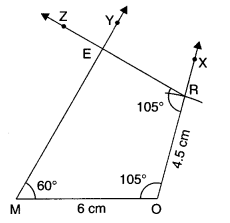3. Cut OR = 4.5 cm from ray OX.
4. At M, draw ray MY such that ∠OMY = 60°.
5. At R, draw ray RZ such that ∠ORZ = 105°.
6. Let the rays MY and RZ meet at E.

Then, MORE is the required quadrilateral.

(ii) Steps of Construction

1. Draw PL = 4 cm.
2. At L, draw ray LX such that ∠PLX = 75°.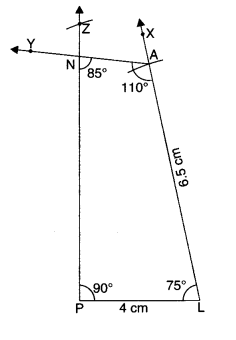By Angle-sum property of a quadrilateral,
∠P + ∠A + ∠N + ∠L = 360°
⇒ 90° + 110° + 85° + Z L = 360°
⇒ 285° + ∠ L = 360°
⇒ ∠L = 360° – 285°
⇒ ∠L = 75°.
3. Cut LA = 6.5 cm from ray LX.
4. At A, draw ray AY such that ∠LAY = 110°.
5. At P, draw ray PZ such that ∠LPZ = 90°.
Let the rays AY and PZ meet at N.

Then, PLAN is the required quadrilateral.

(iii) Steps of Construction

1. Draw HE = 5 cm.
2. At E, draw ray EX such that ∠HEX = 85°
∴ Opposite angles of a parallelogram are equal.
∵ ∠E = ∠R = 85°
3. Cut EA = 6 cm from the ray EX.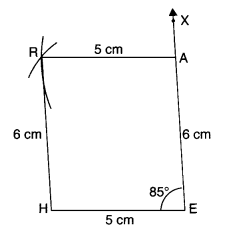4. With A as centre and radius AR = 5 cm, draw an arc.
5. With H as centre and radius HR = 6 cm; draw another arc to intersect the arc drawn in step 4 at R.
∴ opposite sides of a parallelogram are equal in length
∵ AR = EH = 5 cm
and HR = EA = 6 cm
6. Join AR and HR.

Then, HEAR is the required parallelogram.

(iv) Steps of Construction
[We know that each angle of a rectangle
is 90°.
∴ ∠O=∠K=∠A=∠Y= 90°.
Also, opposite sides of a rectangle are equal in length.
∴ OY = KA = 5 cm and AY = KO = 7 cm]

1. Draw OK = 7 cm.
2. At K, draw ray KX such that ∠OKX = 90°.
3. Cut KA – 5 cm from ray KX.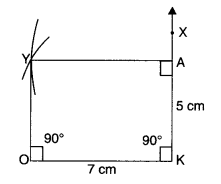4. Taking A as centre and radius AY = 7 cm, draw an arc.
5. Taking O as centre and radius OY = 5 cm, draw another arc to intersect the arc drawn in step 4 at Y.
6. Join AY and OY.

Then OKAY is the required rectangle.

We hope the NCERT Solutions for Class 8 Maths Chapter 4 Practical Geometry Ex 4.3 help you. If you have any query regarding NCERT Solutions for Class 8 Maths Chapter 4 Practical Geometry Ex 4.3, drop a comment below and we will get back to you at the earliest.

## NCERT Solutions for Class 8 Maths Chapter 4 Practical Geometry Ex 4.2

NCERT Solutions for Class 8 Maths Chapter 4 Practical Geometry Ex 4.2 are part of NCERT Solutions for Class 8 Maths. Here we have given NCERT Solutions for Class 8 Maths Chapter 4 Practical Geometry Ex 4.2.

 Board CBSE Textbook NCERT Class Class 8 Subject Maths Chapter Chapter 4 Chapter Name Practical Geometry Exercise Ex 4.2 Number of Questions Solved 1 Category NCERT Solutions

## NCERT Solutions for Class 8 Maths Chapter 4 Practical Geometry Ex 4.2

Question 1.
LI = 4 cm
IF = 3 cm
TL = 2.5 cm
LF = 4.5 cm
IT = 4 cm

OL = 7.5 cm
GL = 6 cm
GD = 6 cm
LD = 5 cm
OD = 10 cm

(iii) Rhombus BEND
BN – 5.6 cm
DE = 6.5 cm
Solution.
(i) Steps of Construction

1. Draw LI = 4 cm.
2. With L as center and radius LT = 2.5 cm, draw an arc.3. With I as center and radius, IT = 4 cm, draw another arc to intersect the arc drawn in step 2 at T.
4. With I as center and radius IF = 3 cm, draw an arc.
5. With L as center and radius LF = 4.5 cm, draw another arc to intersect the arc drawn in step 4 at F.
6. Join IF, FT, TL, LF and IT.

Then, LIFT is the required quadrilateral.

(ii) Steps of Construction

1. Draw LD = 5 cm.
2. With L as center and radius LG = 6 cm, draw an arc.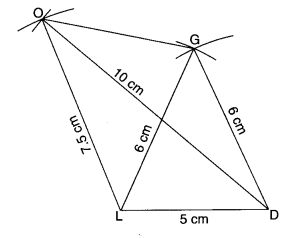3. With D as center and radius DG = 6 cm, draw another arc to intersect the arc drawn in step 2 at G.
4. With L as center and radius LO = 7.5 cm, draw an arc.
5. With D as center and radius DO = 10 cm, draw another arc to intersect the arc drawn in step 4 at O.
6. Join DG, GO, OL, LG and DO.

Then GOLD is the required quadrilateral.

(iii) Steps of Construction

1. Draw DE = 6.5 cm.
2. Draw perpendicular bisector PQ of DE so as to intersect DE at M. Then M is the mid-point of DE.
3. With M as centre and radius
$$=\frac { 1 }{ 2 } \times \left( 5.6 \right) =2.8 cm$$
opposite sides of DE to intersect MP at N and MQ at B.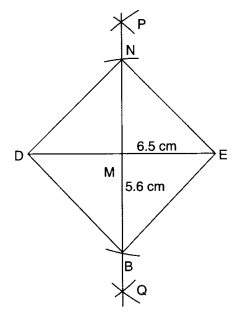4. Join DN, NE, EB, and BD.

Then, BEND is the required rhombus.

We hope the NCERT Solutions for Class 8 Maths Chapter 4 Practical Geometry Ex 4.2 help you. If you have any query regarding NCERT Solutions for Class 8 Maths Chapter 4 Practical Geometry Ex 4.2, drop a comment below and we will get back to you at the earliest.

## NCERT Solutions for Class 8 Maths Chapter 3 Understanding Quadrilaterals Ex 3.4

NCERT Solutions for Class 8 Maths Chapter 3 Understanding Quadrilaterals Ex 3.4 are part of NCERT Solutions for Class 8 Maths. Here we have given NCERT Solutions for Class 8 Maths Chapter 3 Understanding Quadrilaterals Ex 3.4.

 Board CBSE Textbook NCERT Class Class 8 Subject Maths Chapter Chapter 3 Chapter Name Understanding Quadrilaterals Exercise Ex 3.4 Number of Questions Solved 6 Category NCERT Solutions

## NCERT Solutions for Class 8 Maths Chapter 3 Understanding Quadrilaterals Ex 3.4

Question 1.
State whether True or False :
(a) All rectangles are squares
(b) All rhombuses are parallelograms
(c) All squares are rhombuses and also rectangles
(d) All squares are not parallelograms
(e) All kites are rhombuses
(f) All rhombuses are kites
(g) All parallelograms are trapeziums
(h) All squares are trapeziums.
Solution.
(b), (c), (f), (g), (h) are true;
others are false.

Question 2.
Identify all the quadrilaterals that have.
(a) four sides of equal length
(b) four right angles
Solution.
(a) Rhombus; square
(b) Square; rectangle

Question 3.
Explain how a square is
(ii)a parallelogram
(iii) a rhombus
(iv) a rectangle.
Solution.
A square is 4 sided, so it is a quadrilateral.

(ii) a parallelogram
A square has its opposite sides parallel; so it is a parallelogram.

(iii) a rhombus
A square is a parallelogram with all the 4 sides equal, so it is a rhombus.

(iv) a rectangle
A square is a parallelogram with each angle a right angle; so it is a rectangle.

Question 4.
Name the quadrilaterals whose diagonals :
(i) bisect each other
(ii) are perpendicular bisectors of each other
(iii) are equal.
Solution.
(i) bisect each other
The names of the quadrilaterals whose diagonals bisect each other are parallelogram; rhombus; square; rectangle.

(ii) are perpendicular bisectors of each other
The names of the quadrilaterals whose diagonals are perpendicular bisectors of each other are rhombus; square.

(iii) are equal
The names of the quadrilaterals whose diagonals are equal are square; rectangle.

Question 5.
Explain why a rectangle is a convex quadrilateral.
Solution.
A rectangle is a convex quadrilateral because both of its diagonals lie wholly in its interior.

Question 6.
ABC is a right-angled triangle and O is the mid-point of the side opposite to the right angle. Explain why O is equidistant from A, B and C. (The dotted lines are drawn additionally to help you).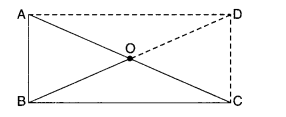Solution.
Construction: Produce BO to D such that BO = OD. Join AD and CD.
Proof. AO = OC ∵ O is the mid-point of AC
BO = OD By construction
∴ Diagonals of quadrilateral ABCD bisect each other.
∴ Quadrilateral ABCD is a parallelogram.
Now, ∠ABC = 90° given
∴ ABCD is a rectangle.
Since the diagonals of a rectangle bisect each other, therefore,
O is the mid-point of AC and BD both. But AC = BD
∵ Diagonals of a rectangle are equal
∴ OA = OC =$$\frac { 1 }{ 2 }$$AC =$$\frac { 1 }{ 2 }$$BD = OB
⇒ OA = OB = OC.

We hope the NCERT Solutions for Class 8 Maths Chapter 3 Understanding Quadrilaterals Ex 3.4 help you. If you have any query regarding NCERT Solutions for Class 8 Maths Chapter 3 Understanding Quadrilaterals Ex 3.4, drop a comment below and we will get back to you at the earliest.

## NCERT Solutions for Class 8 Maths Chapter 3 Understanding Quadrilaterals Ex 3.3

NCERT Solutions for Class 8 Maths Chapter 3 Understanding Quadrilaterals Ex 3.3 are part of NCERT Solutions for Class 8 Maths. Here we have given NCERT Solutions for Class 8 Maths Chapter 3 Understanding Quadrilaterals Ex 3.3.

 Board CBSE Textbook NCERT Class Class 8 Subject Maths Chapter Chapter 3 Chapter Name Understanding Quadrilaterals Exercise Ex 3.3 Number of Questions Solved 12 Category NCERT Solutions

## NCERT Solutions for Class 8 Maths Chapter 3 Understanding Quadrilaterals Ex 3.3

Question 1.
Given a parallelogram ABCD. Complete each statement along with the definition with the definition or property used.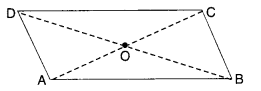(ii) ∠DCB = …………….
(iii) OC = ……………….
(iv) m∠DAB + m∠CDA = …………..
Solution.
Opposite sides of a parallelogram are equal

(ii) ∠DCB = ∠DAB
Opposite angles of a parallelogram are equal

(iii) OC = OA
∵ Diagonals of a parallelogram bisect each other

(iv) m∠DAB + m∠CDA = 180°
∵ Adjacent angles of a parallelogram are supplementary.

Question 2.
Consider the following parallelo¬grams. Find the values of the unknowns x, y, z.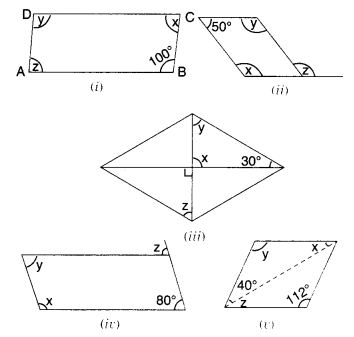Solution.
(i) y = 100°
Opposite angles of a parallelogram are equal
x + 100° = 180°
Adjacent angles in a parallelogram are supplementary
⇒ x = 180° – 100°
⇒ x = 80°
⇒ z – x = 80°
Opposite angles of a parallelogram are of equal measure

(ii) x + 50° = 180°
Adjacent angles in a parallelogram are supplementary
⇒ x = 180° – 50° = 130°
⇒ y = x = 130°
The opposite angles of a parallelogram are of equal measure
180° – z = 50°
Opposite angles of a parallelogram are of equal measure
⇒ z = 180° – 50° = 130°

(iii) x = 90°
Vertically opposite angles are equal
x + y + 30° = 180°
By angle sum property of a triangle
⇒ 90° + y + 30° = 180°
⇒ 120° + y = 180°
⇒ y = 180° – 120° = 60° z + 30° + 90° – 180°
By angle sum property of a triangle
z = 60°

(iv) y = 80°
Opposite angles of a parallelogram are of equal measure
x + 80° = 180°
Adjacent angles in a parallelogram are supplementary
⇒ x = 180° – 80°
⇒ x = 100°
⇒ 180°-2+ 80°= 180°
Linear pair property and adjacent angles in a parallelogram are supplementary.
z = 80°

(v) y = 112°
Opposite angles of a parallelogram are equal
x + y + 40° = 180°
By angle sum property of a triangle
⇒ x + 112° + 40° = 180°
⇒ x + 152° = 180°
⇒ x = 180°- 152°
⇒ x = 28°
z = x = 28°.
Alternate interior angles

Question 3.
Can a quadrilateral ABCD be a parallelogram if
(i) ∠D + ∠B = 180° ?
(ii) AB = DC = 8 cm, AD = 4 cm and BC = 4.4 cm
(iii) ∠A = 70° and ∠C = 65°?
Solution.
(i) Can be, but need not be
(ii) No: in a parallelogram, opposite sides are equal; but here, AD ≠ BC.
(iii) No: in a parallelogram, opposite angles are of equal measure; but here ∠A ≠ ∠C.

Question 4.
Draw a rough figure of a quadrilateral that is not a parallelogram but has exactly two opposite angles of equal measure.
Solution.
A kite, for exampleQuestion 5.
The measures of two adjacent angles of a parallelogram are in the ratio 3:2. Find the measure of each of the angles of the parallelogram.
Solution.
Let the two adjacent angles be 3x° and 2x°.
Then,
3x° + 2x° = 180°
∴ Sum of the two adjacent angles of a parallelogram is 180°
⇒ 5x° = 180°
⇒ $${ x }^{ \circ }=\frac { { 180 }^{ \circ } }{ 5 }$$
⇒ x° = 36°
⇒ 3x° = 3 x 36° = 108°
and
2x° = 2 x 36° = 72°.
Since, the opposite angles of a parallelogram are of equal measure, therefore the measures of the angles of the parallelogram are 72°, 108°, 72°, and 108°.

Question 6.
Two adjacent angles of a parallelogram have equal measure. Find the measure of each of the angles of the parallelogram.
Solution.
Let the two adjacent angles of a parallelogram be x° each.
Then,
x° + x° = 180°
∴ Sum of the two adjacent angles of a parallelogram is 180°.
⇒ 2x° = 180°
⇒ $${ x }^{ \circ }=\frac { { 180 }^{ \circ } }{ 2 }$$
⇒ x° = 90°.
Since the opposite angles of a parallelogram are of equal measure, therefore the measure of each of the angles of the parallelogram is 90°, i.e., each angle of the parallelogram is a right angle.

Question 7.
The adjacent figure HOPE is a parallelogram. Find the angle measures x, y and z. State the properties you use to find them.
Solution.
x = 180° – 70° = 110°
Linear pair property and the opposite angles of a parallelogram are of equal measure.
∵ HOPE is a || gm
∴ HE || OP
and HP is a transversal
∴ y = 40°
alternate interior angles
40° + z + x = 180°
The adjacent angles in a parallelogram are supplementary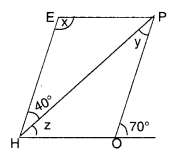⇒ 40° + z + 110° = 180°
⇒ z + 150° = 180°
⇒ z = 180° – 150°
⇒ z = 30°.

Question 8.
The following figures GUNS and RUNS are parallelograms. Find x and y. (Lengths are in cm)
(i)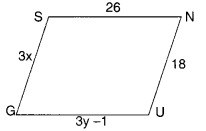(ii)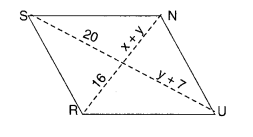Solution.
(i)
For Figure GUNS
Since the opposite sides of a parallelogram are of equal length, therefore,
⇒ 3x = 18
⇒ $$x=\frac { 18 }{ 3 } =6$$
and, 3y – 1 = 26
⇒ 3y = 26 + 1
⇒ 3y = 27
$$y=\frac { 27 }{ 3 } =9$$
Hence, x = 6; y = 9.

(ii)
For Figure RUNS
Since the diagonals of a parallelogram bisect each other, therefore,
⇒ x + y = 16 …(1)
and, y + 7 = 20 …(2)
From (2),
⇒ y – 20 – 7 = 13
Putting y = 13 in (1), we get
⇒ x + 13 = 16 ⇒ x = 16 – 13 = 3.
Hence, x = 3; y = 13.

Question 9.
In the below figure both RISK and CLUE are parallelograms. Find the value of x.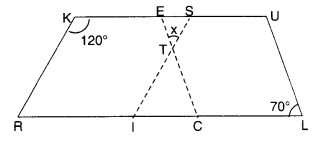Solution.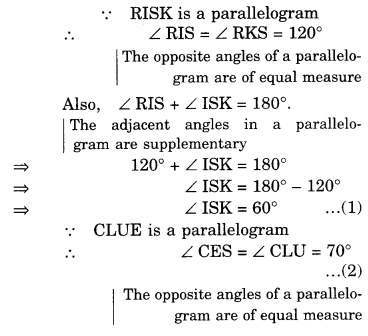Question 10.
Explain how this figure is a trapezium. Which of its two sides is parallel?
Solution.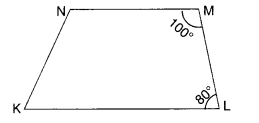∵ ∠KLM + ∠NML = 80° + 100° = 180°
∴ KL || NM
∵ The sum of consecutive interior angles is 180°
∴ Figure KLMN is a trapezium.
Its two sides $$\overline { KL }$$ and $$\overline { NM }$$ are parallel.

Question 11.
Find m∠C in the figure, if $$\overline { AB }$$ || $$\overline { DC }$$.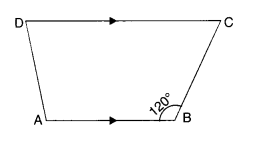Solution.
∵ $$\overline { AB }$$ || $$\overline { DC }$$
∴ m∠C + m∠B = 180°
∵ The sum of consecutive interior angles is 180°
m∠C+ 120° = 180°
⇒ m∠C = 180° – 120° = 60°.

Question 12.
Find the measure of ∠P and ∠S, if $$\overline { SP }$$ || $$\overline { RQ }$$ in the figure. (If you find mZ R, is there more than one method to find m∠P ?)
Solution.∵ $$\overline { SP }$$ || $$\overline { RQ }$$
∴ m∠P+m∠Q = 180°
∵ The sum of consecutive interior angles is 180°
⇒ m∠P + 130° = 180°
⇒ m∠P = 180° – 130°
⇒ m∠P = 50°
Again, m∠R + m∠S = 180°
∵ The sum of consecutive interior angles is 180°
⇒ 90° + m Z S = 180°
⇒ m∠S = 180° – 90° = 90°
Yes; there is one more method of finding m∠P if m∠R is given and that is by using the angle sum property of a quadrilateral.
We have,
m∠P + m∠Q + m∠R + m∠S = 360°
⇒ m∠P + 130° + 90° + 90° = 360°
⇒ m∠P + 310° = 360°
⇒ m∠P = 360° – 310° = 50°.

We hope the NCERT Solutions for Class 8 Maths Chapter 3 Understanding Quadrilaterals Ex 3.3 help you. If you have any query regarding NCERT Solutions for Class 8 Maths Chapter 3 Understanding Quadrilaterals Ex 3.3, drop a comment below and we will get back to you at the earliest.

## NCERT Solutions for Class 8 Maths Chapter 3 Understanding Quadrilaterals Ex 3.2

NCERT Solutions for Class 8 Maths Chapter 3 Understanding Quadrilaterals Ex 3.2 are part of NCERT Solutions for Class 8 Maths. Here we have given NCERT Solutions for Class 8 Maths Chapter 3 Understanding Quadrilaterals Ex 3.2.

 Board CBSE Textbook NCERT Class Class 8 Subject Maths Chapter Chapter 3 Chapter Name Understanding Quadrilaterals Exercise Ex 3.2 Number of Questions Solved 6 Category NCERT Solutions

## NCERT Solutions for Class 8 Maths Chapter 3 Understanding Quadrilaterals Ex 3.2

Question 1.
Find x in the following figures.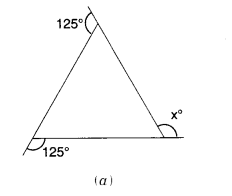Solution.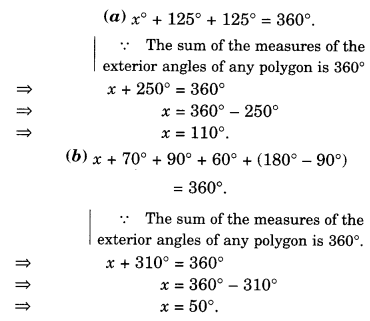Question 2.
Find the measure of each exterior angle of a regular polygon of
(i) 9 sides
(ii) 15 sides
Solution.
(i) 9 sides
Measure of each exterior angle=$$\frac { { 360 }^{ \circ } }{ 9 } ={ 40 }^{ \circ }$$

(ii) 15 slides
Measure of each exterior angle=$$\frac { { 360 }^{ \circ } }{ 15 } ={ 24 }^{ \circ }$$

Question 3.
How many sides does a regular polygon have if the measure of an exterior angle is 24°?
Solution.
Let the number of sides be n. Then, n(24°)=360°.
⇒ $$n=\frac { { 360 }^{ \circ } }{ 24 } =15$$
Hence, the number of sides is 15.

Question 4.
How many sides does a regular polygon have if each of its interior angles is 165°?
Solution.
∵ Each interior angle=165°
∴ Each exterior angle
= 180°-165°=15°
linear pair property
Let the number of sides be n. Then,
n(15°)=360°
$$n=\frac { { 360 }^{ \circ } }{ { 15 }^{ \circ } } =24$$
Hence, the number of sides is 24.

Question 5.
(a) Is it possible to have a regular polygon with a measure of each exterior angle at 22°?
(b) Can it be an interior angle of a regular polygon? Why?
Solution.
(a) No ; (since 22 is not a factor of 360).
(b) No ; (because each exterior angle is 180° – 22° = 158°, which is not a factor of 360°).

Question 6.
(a) What is the minimum interior angle possible for a regular polygon? Why?
(b) What is the maximum exterior angle possible for a regular polygon?
Solution.
(a) The equilateral triangle is a regular polygon of 3 sides has the minimum measure of an interior angle = 60°.
(b) By (a), we can see that the maximum exterior angle possible for a regular polygon is 180° – 60° = 120°.

We hope the NCERT Solutions for Class 8 Maths Chapter 3 Understanding Quadrilaterals Ex 3.2 help you. If you have any query regarding NCERT Solutions for Class 8 Maths Chapter 3 Understanding Quadrilaterals Ex 3.2, drop a comment below and we will get back to you at the earliest.